# Quiz 6: Transportation, Transshipment, and Assignment Problems

Business

Consider the following table as shown below: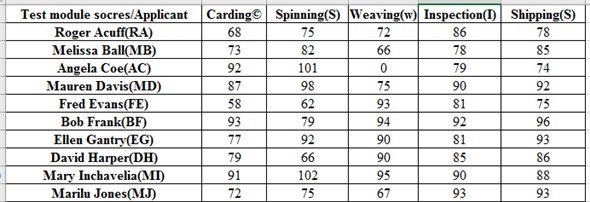Thus, the assignment problem is unbalance one i.e. number of rows is not equal to number of columns. So, one should add 5 new columns to balance the problem.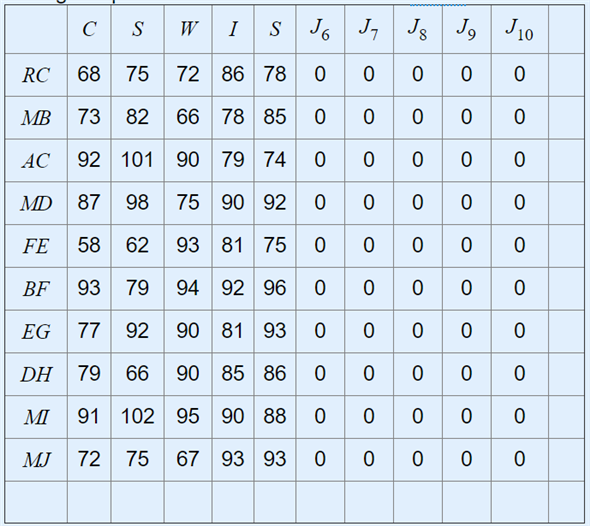Hence, now it is balanced assignment problem i.e. number of rows is equal to number of columns. Step 1: Identify the least element for each row and subtract the least element from every element of the particular row as shown below: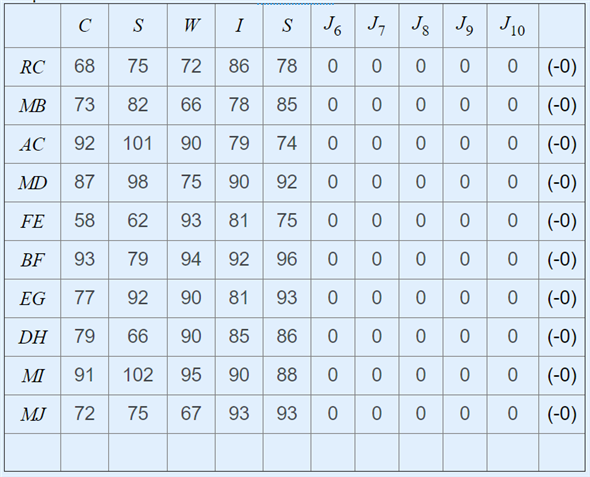Step2: Identify the least element for each column and subtract the least element from every element of the particular column as shown below: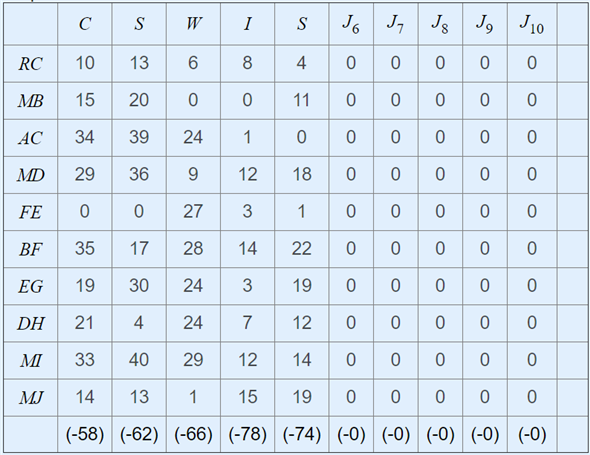Step3: Now, one can observe that each row and each column has a single zero starting from first row enrectangle a single row and cross-of all other zeroes in the particular row and in the corresponding column.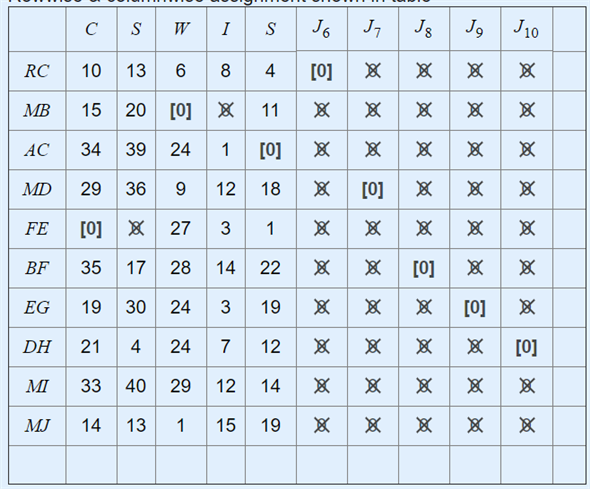Step4: Thus, from the above it is clear that the number of assignments is not equal to the order of the matrix (i.e. n=10). • Mark a row which doesn't have any assignment. • In the marked row mark a column which has a crossed zero. • In the marked row mark a row which has assignment. • Draw dotted lines through the marked column and unmarked rows. Thus, the elements which are not covered by the dotted lines are called uncovered elements.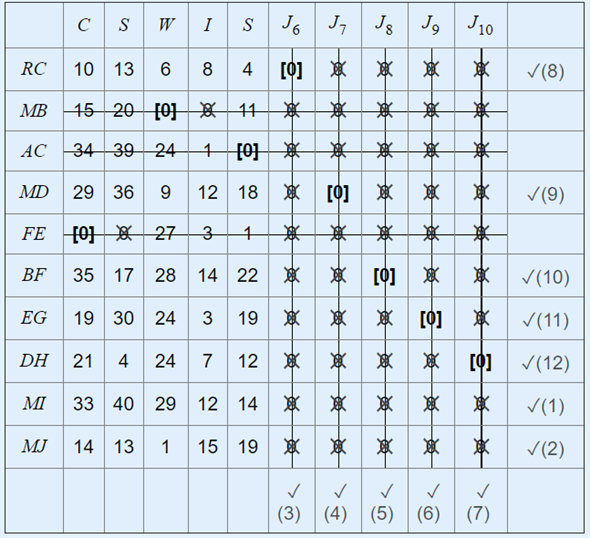Step 5: Now, among the entire uncovered elements one should identify the least elements from the above table and subtract the least element from all the uncovered elements.Add the least uncovered elements wherever the element is intersected by the dotted lines. Here, the least element is 1.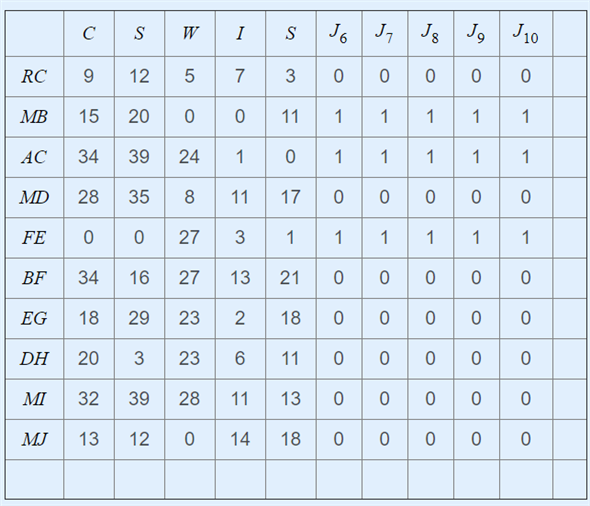Step6: Now, in order to obtain an optimal solution repeat steps 3 to 5 as shown below Now, again one can observe that each row and each column has a single zero starting from first row enrectangle a single row and cross-of all other zeroes in the particular row and in the corresponding column.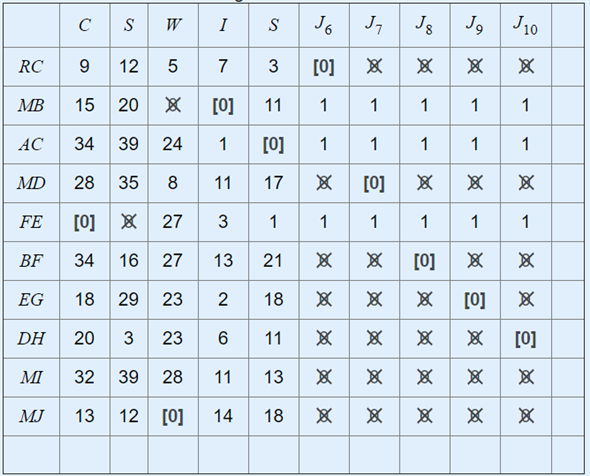Step7: Thus, from the above it is clear that the number of assignments is not equal to the order of the matrix (i.e. n=10). • Mark a row which doesn't have any assignment. • In the marked row mark a column which has a crossed zero. • In the marked row mark a row which has assignment. • Draw dotted lines through the marked column and unmarked rows. Thus, the elements which are not covered by the dotted lines are called uncovered elements.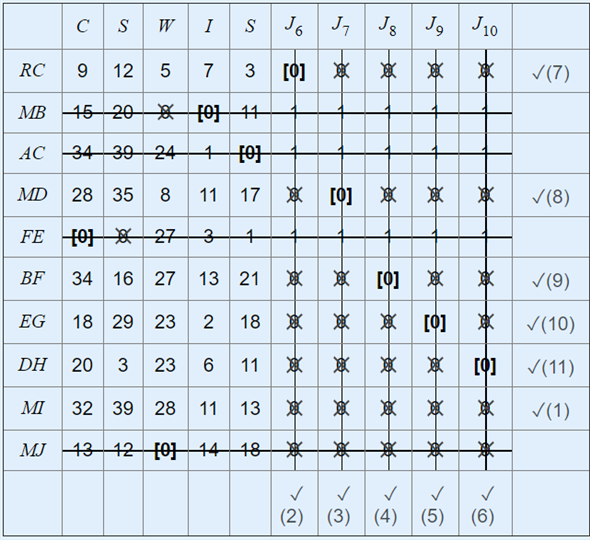Step8: Now, among the entire uncovered elements one should identify the least elements from the above table and subtract the least element from all the uncovered elements.Add the least uncovered elements wherever the element is intersected by the dotted lines. Here, the least element is 2.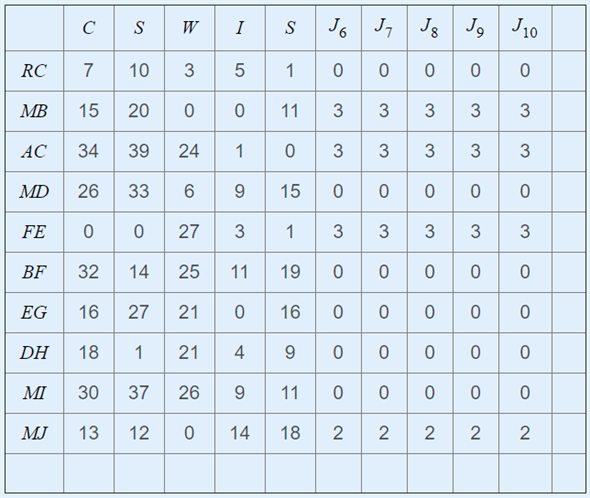Step9: Now, in order to obtain an optimal solution repeat steps 3 to 5 as shown below Now, again one can observe that each row and each column has a single zero starting from first row enrectangle a single row and cross-of all other zeroes in the particular row and in the corresponding column.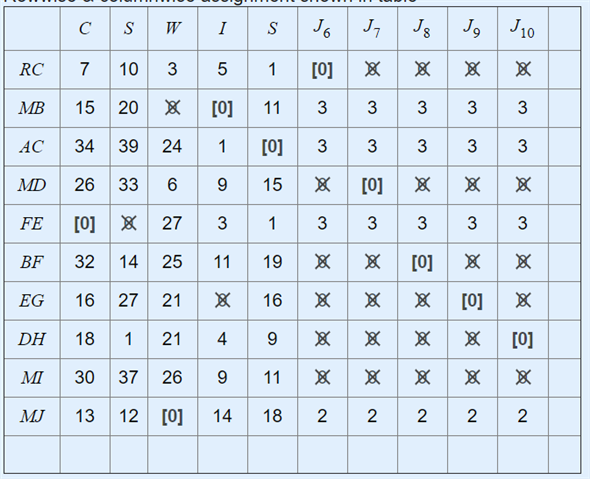Step10: Thus, from the above it is clear that the number of assignments is not equal to the order of the matrix (i.e. n=10). • Mark a row which doesn't have any assignment. • In the marked row mark a column which has a crossed zero. • In the marked row mark a row which has assignment. • Draw dotted lines through the marked column and unmarked rows. Thus, the elements which are not covered by the dotted lines are called uncovered elements.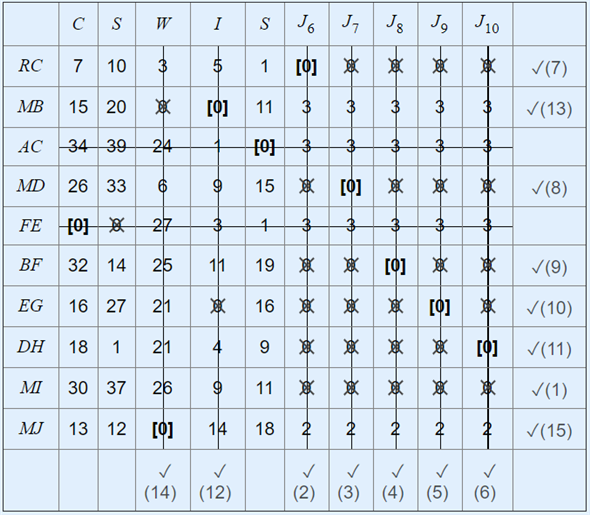Step11: Now, among the entire uncovered elements one should identify the least elements from the above table and subtract the least element from all the uncovered elements. Add the least uncovered elements wherever the element is intersected by the dotted lines. Here, the least element is 1.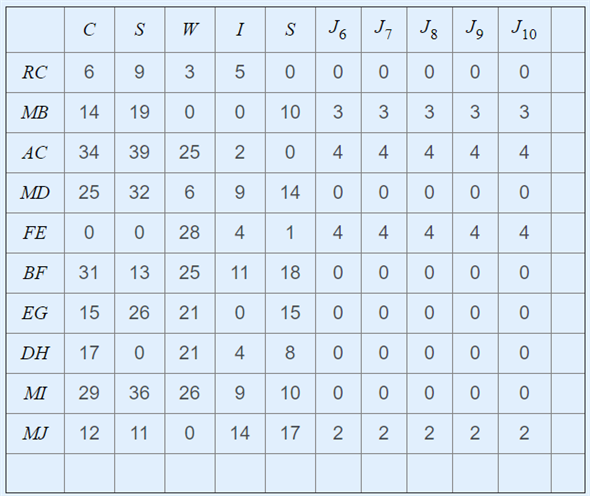Step12: Now, in order to obtain an optimal solution repeat steps 3 to 5 as shown below Now, again one can observe that each row and each column has a single zero starting from first row enrectangle a single row and cross-of all other zeroes in the particular row and in the corresponding column.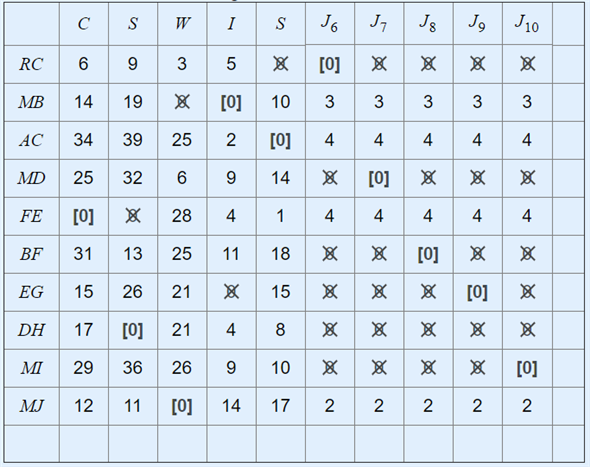Step 13: Thus, from the above it is clear that the number of assignments is equal to the order of the matrix (i.e. n=10). Therefore; the solution is optimal as shown above. The assignment schedule for B is as follows: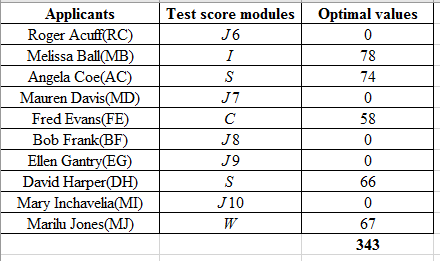Therefore, optimal solution for B is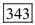.

Person E needs to determine the least costly shipments of materials and jackets that would meet the requirements of Country U distribution centers. Prepare and solve the linear programming model using excel spreadsheet as shown below: Prepare a spreadsheet using the following values and formulas: Figure 1: Screenshot of formulas used in spreadsheet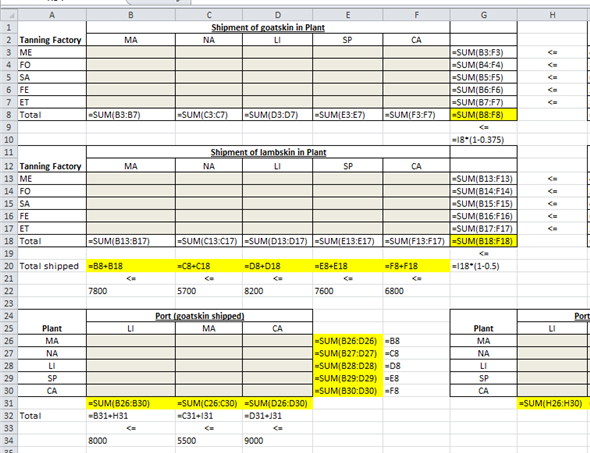Figure 2: Screenshot of formulas used in spreadsheet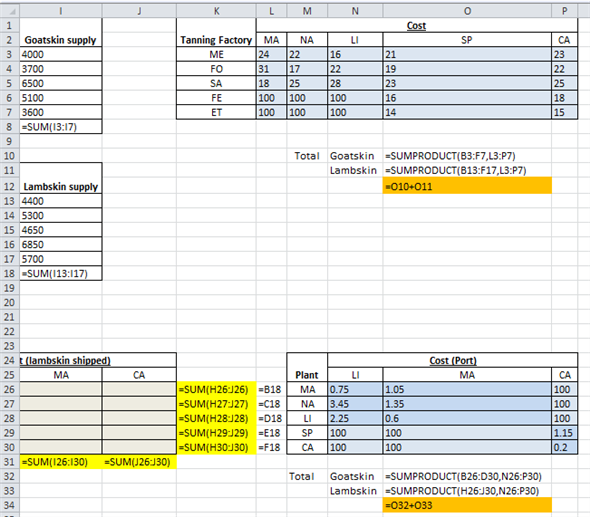Figure 3: Screenshot of formulas used in spreadsheet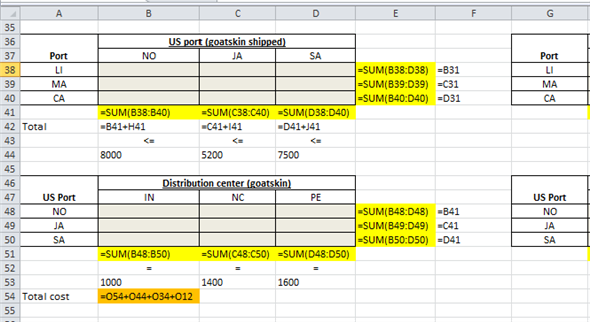Figure 4: Screenshot of formulas used in spreadsheet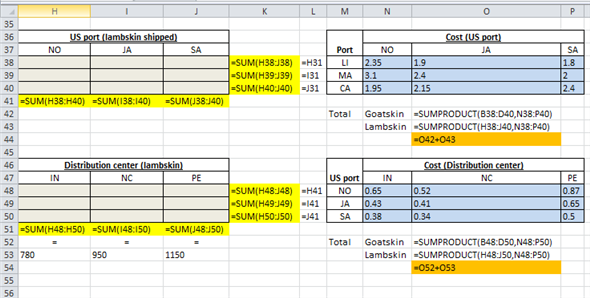The formulated excel spreadsheet is shown below: Figure 5: Screenshot of formulated spreadsheet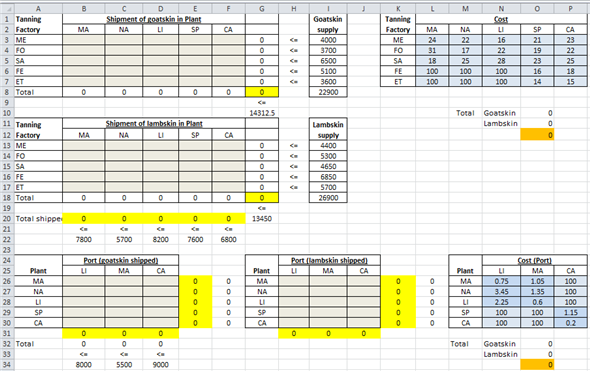Figure 6: Screenshot of formulated spreadsheet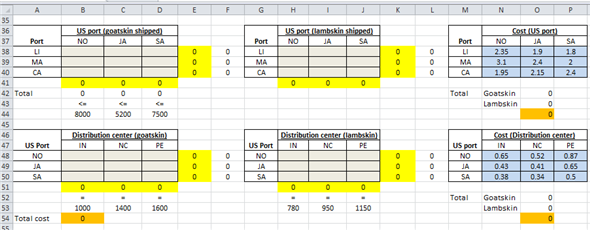Now, click on excel "Solver" option. Put the following values in the "Solver" window: Figure 7: Screenshot of Excel solver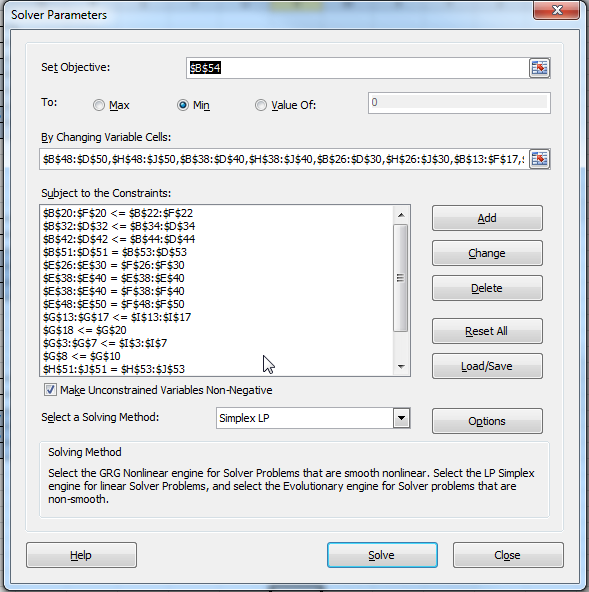Figure 8: Screenshot of Excel solver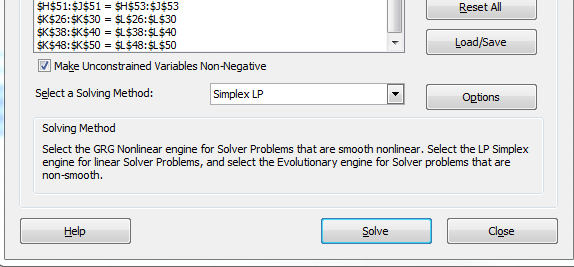Click on "Solve" option. Then, click on "OK" option. It would give the following results: Figure 9: Screenshot of final results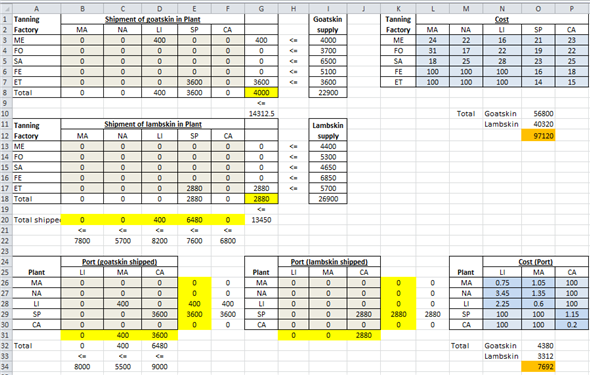Figure 10: Screenshot of final results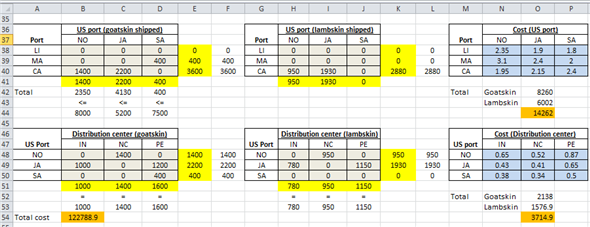Hence, the optimal transhipment model is obtained for the company that would provide a least costly shipments of material and jackets. The minimum cost involved in this model is \$122,788.90.

First, put together the linear programming model; where the destinations are represented with the numbers 1-4 and the sources are 1-3. For this problem, we will use A, B, C and D to represent the destinations. Consider the following table: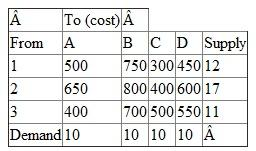MaximizeThis would be subject to the following equationsRepresenting the supplyRepresenting the demand Where,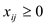Now, to solve this transportation problem, use the QM for Window software (provided with the book) following these instructions: First, open the software; under module, select Transportation. Next, open a blank document. A menu will open; enter the document title if needed. Then, enter the number of Sources (1, 2 and 3) which is 3 for this problem. Enter the number of destinations which is 4 for this problem ( A, B, C and D ). Select OK and a window will show in order to enter the equations. Enter the values for Destination and Sources; and enter the given supply and demand numbers (3 and 4 for this problem); then, select solve on the top right corner and a series of windows with solutions. The window titled: Transportation Shipments has the values of the variables. From source 1 to destination C the value is 2 From source 1 to destination D the value is 10 From source 2 to destination B the value is 9 From source 2 to destination C the value is 8 From source 3 to destination A the value is 10 From source 3 to destination B the value is 1 From source 1 to destination A the value is 0 Hence,There is no answer for this question